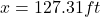## Will give brainliest and extra points!! Aurora hit a baseball with an initial velocity of 70 feet per second at an angle of 30° with

Question

Will give brainliest and extra points!!
Aurora hit a baseball with an initial velocity of 70 feet per second at an angle of 30° with the horizontal. The ball hit her bat when the ball was 3 feet above the ground.

(a) No one interferes with the ball. How long does it take the ball to hit the ground? Round your answer to the nearest hundredth of a second. Show all your work.

in progress 0
5 months 2021-09-05T16:56:26+00:00 1 Answers 2 views 0

1. Step-by-step Explanation:

Given: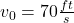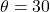Let’s consider the point where the baseball was hit to be the origin of our coordinate system. The equation for the y-motion of the baseball can be written as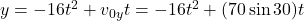or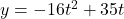a) In order to find the time of flight of the baseball, we know that it will hit the ground when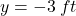so we can rewrite the equation for as follows: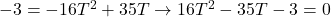where T is the time of flight. Solving for the roots of the quadratic equation, we get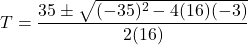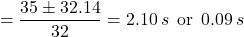We take the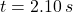as the time it takes for the baseball to hit the ground.

b) We know that the horizontal distance traveled by the baseball is given by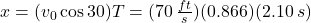or